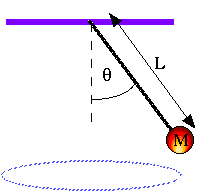# Tension + Centripetal Force

## Homework StatementThe pendulum is swinging at an angle of 25.5 degrees (with respect to the vertical)
The mass of the object is 14.6 kg
Length of String is .5 m
Find the velocity (v = ?)

## Homework Equations

Centripetal Acceleration (ac) = v^2/r
Centripetal Force (Fc) = m*ac

## The Attempt at a Solution

I'm getting stuck at this problem after a certain point. I've found the radius of the circle using the right angle trig identities.
R = .215 m

I also know that the problem has to do with the sum of the forces of Fcp, Tension, and Force due to Gravity.
The y-component of the forces should be 0, so Fg (force of gravity) minus y component of Tension equals 0.

Therefore, I know that Fg = 143.08, and the y-component of Tension = 143.08.
However, I'm having trouble figuring out what to do for the x-component of the forces, so that I can use it to find the velocity.

## Answers and Replies

What would the tangent of the angle tell you? If you're solving for "v" in the centripetal force equation, keep in mind this is the speed of the ball rather than the velocity, in which case we need not consider x and y components.

u need set up the equations into two parts x, y

the x component of this pendulum would be
Fx = ma = Tx-mr^2/R = 0
where Tx is your x-component of the tension
the sum of the horizontal forces must equal to zero in order for the ball to move in the circle

Fy = ma = Ty-mg = 0
the sum of the veritcal forces must equal to zero in order for the ball to stay on the string

Doc Al
Mentor
conical pendulum

## The Attempt at a Solution

I'm getting stuck at this problem after a certain point. I've found the radius of the circle using the right angle trig identities.
R = .215 m
Good.
I also know that the problem has to do with the sum of the forces of Fcp, Tension, and Force due to Gravity.
Careful here. Only two forces act on the mass: Weight and string tension. (Centripetal force is not a separate force, just the name given to any force that creates a centripetal acceleration.)
The y-component of the forces should be 0, so Fg (force of gravity) minus y component of Tension equals 0.
Good.

Therefore, I know that Fg = 143.08, and the y-component of Tension = 143.08.
Since you know the angle of the string, you also know the full value of the tension. But you don't really need to calculate it. (See below.)
However, I'm having trouble figuring out what to do for the x-component of the forces, so that I can use it to find the velocity.
Apply Newton's 2nd law. For the x-direction, the acceleration is not zero.

Set up your two force equations (for x and y) and combine them to solve for v.

Hint: Express the x and y components of the tension as $T\sin\theta$ and $T\cos\theta$. There's no need to calculate T (unless they ask for it).

Thanks. I ended up finding the x-component of the Tension Force, and used it to find the acceleration in the x-direction. From there I used the centripetal acceleration to get about 1.004 m/s for the speed.